Courses

# RD Sharma Solutions -Page No.9.14, Ratio Proportion And Unitary Method, Class 6, Maths Class 6 Notes | EduRev

## RD Sharma Solutions for Class 6 Mathematics

Created by: Abhishek Kapoor

## Class 6 : RD Sharma Solutions -Page No.9.14, Ratio Proportion And Unitary Method, Class 6, Maths Class 6 Notes | EduRev

The document RD Sharma Solutions -Page No.9.14, Ratio Proportion And Unitary Method, Class 6, Maths Class 6 Notes | EduRev is a part of the Class 6 Course RD Sharma Solutions for Class 6 Mathematics.
All you need of Class 6 at this link: Class 6

PAGE NO 9.14:

Question 1: Which of the following statements are true?

(i) 16 : 24 = 20 : 30

(ii) 21 : 6 = 35 : 10

(iii) 12 : 18 = 28 : 12

(iv) 51 : 58 = 85 : 102

(v) 40 men : 200 men = Rs 5 : Rs 25

(vi) 99 kg : 45 kg = Rs 44 : Rs 20

ANSWER: (i) 16 : 24 = 20 : 30

16/24 = 2/3 (Dividing numerator and denominator by 8)

20/30 = 2/3 (Dividing numerator and denominator by 10)

∴ 16/24 = 20/30

Thus, the statement is true.

(ii) 21 : 6 = 35 : 10

21/6 = 7/2 (Dividing numerator and denominator by 3)

35/10 = 7/2 (Dividing numerator and denomenator by 5)

∴ 21/6 = 35/10

Thus, the statement is true.

(iii) 12 : 18 = 28 : 12

12/18 = 2/3 (Dividing numerator and denominator by 6)

28/12 = 7/3 (Dividing numerator and denominator by 4)

∴ 16/24 = 20/30 are not equal.

Thus, the statement is not true.

(iv) 51 : 58 = 85 : 102

51/58

And 85/102 = 5/6 (Dividing numerator and denominator by 17)

51/58  is not equal to 85/102.

Thus, the statement is not true.

(v) 40 men : 200 men = Rs 5 : Rs 25

40/200 = 1/5 (Dividing numerator and denominator by 40)

5/25 = 1/5 (Dividing numerator and denominator by 5)

∴ 40/200 = 5/25

Thus, the statement is true.

(vi) 99 kg : 45 kg = Rs 44 : Rs 20

99/45 = 11/5 (Dividing numerator and denominator by 9)

44/20 = 11/5 (Dividing numerator and denominator by 4)

∴ 99/45 = 11/5

Thus, the statement is true.

Question 2: Find which of the following are in proportion:

(i) 8, 16, 6, 12
(ii) 6, 2, 4, 3
(iii) 150, 250, 200, 300

ANSWER: (i) Consider 8/16 = 1/2

And 6/12 = 1/2

∵ 8 : 16 = 6 : 12

∴ 8, 16, 6, 12 are in proportion.

(ii) Consider  6/2 = 3/1

And 4/3

∵  6 : 2 ≠≠  4 : 3
∴ 6, 2, 4, 3 are not in proportion.

(iii) Consider  150/250 = 3/5 (Dividing numerator and denominator by 50)

And 200/300 = 2/3  (Dividing numerator and denominator by 100)

∵ 150 : 250 ≠≠  200 : 300

∴ 150, 250, 200, 300 are not in proportion.

Question 3: Find x in the following proportions:

(i) x : 6 = 55 : 11

(ii) 18 : x = 27 : 3

(iii) 7 : 14 = 15 : x

(iv) 16 : 18 = x : 96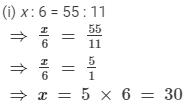(ii) 18 : x = 27 : 3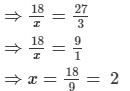(iii) 7 : 14 = 15 : x1624=23 (Dividing numerator and denominator by 8)2030= 23 (Dividing numerator and denominator by 1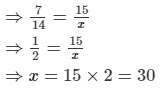(iv) 16 : 18 = x : 96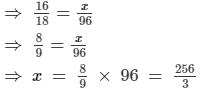Question 4: Set up all proportions from the numbers 9, 150, 105, 1750.

All proportions are:
9 : 150 = 3 : 50
9 : 105 = 3 : 35
9 : 1750
150 : 105 = 10 : 7
150 : 1750 = 3 : 35
105 : 1750 = 3 : 50
Thus, all proportions that can be formed are:
3 : 50, 3 : 35, 10 : 7, 9 : 1750, 1750 : 9, 7 : 10, 35 : 3 and 50 : 3

Question 5:

Find the other three proportions involving terms of each of the following:
(i) 45 : 30 = 24 : 16
(ii) 12 : 18 = 14 : 21

ANSWER: (i) 45 : 30 = 24 : 16  (3 : 2 is its simplest form)
Other three proportions are:
45 : 24 = 30 : 16  (15 : 8 is its simplest form)
30 : 45 = 16 : 24  (2 : 3 is its simplest form)
16 : 30 = 24 : 45  (8 : 15 is its simplest form)
(ii) 12 : 18 = 14 : 21 (2 : 3 is its simplest form)
Other three proportions are:
12 : 14 = 18 : 21 (6 : 7 is its simplest form)
21 : 18 = 14 : 12 (7 : 6 is its simplest form)
18 : 12 = 21 : 14 (3 : 2 is its simplest form)

Question 6: If 4, x, 9 are in continued proportion, find the value x.

ANSWER: It is given that 4, x, 9 are in continued proportion; therefore, we have:
4 : x : : x : 9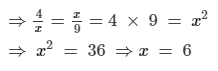Question 7: If in a proportion, the first, second and fourth terms are 32, 112 and 217 respectively, find the third term.

ANSWER: In a proportion, the first, second and fourth terms are 32, 112 and 217, respectively.

Let the third term is be x.

Then, we have:

32 : 112 : : x : 217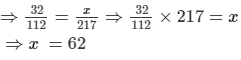Question 8: Show that the following numbers are in continued proportion:
(i) 36, 90, 225
(ii) 48, 60, 75
(iii) 16, 84, 441

Consider 36/90 = 2/5  (Dividing numerator and denominator by 18)

90/225 = 2/5   (Dividing numerator and denominator by 45)

36 : 90 : : 90 : 225
(ii) 48, 60, 75
Consider  48/60 = 4/5 (Dividing numerator and denominator by 12)

60/75 = 4/5  (Dividing numerator and denominator by 15)

⇒ 48 : 60 : : 60 : 75
(iii) 16, 84, 441
Consider 16/84 = 4/21 (Dividing numerator and denominator by 4)

84/441 = 4/21 (Dividing numerator and denominator by 4)

⇒ 16 : 84 : : 84 : 441

Question 9: The ratio of the length of a school ground to its width is 5 : 2. Find its length if the width is 40 metres.

ANSWER: Ratio of the length to the width of a school ground = 5 : 2

∵ Width = 40 m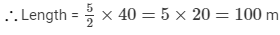Question 10: The ratio of the sale of eggs on  a Sunday to that of the whole week of a grocery shop was 2 : 9. If the total sale of eggs in the same week was Rs 360, find the sale of eggs on Sunday.

ANSWER: Ratio of the sale of eggs on Sunday to that of the whole week = 2 : 9
When total eggs of Rs. 9 is sold in a week, the sale of eggs on Sunday = Rs. 2
When total eggs of Rs. 1 sold in a week, th sale of eggs on Sunday = 2/9

When total eggs of Rs. 360 sold in a week, the sale of eggs on Sunday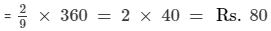Question 11: The ratio of copper and zinc in an alloy is 9 : 7. If the weight of zinc in the alloy is 9.8 kg, find the weight of copper in the alloy.

ANSWER: The ratio of copper and zinc in an alloy is 9 : 7.
When the weight of zinc is 7 kg, the weight of copper = 9 kg

When the weight of zinc is 1 kg, the weight of copper  = 9/7 kg

When the weight of zinc is 9.8 kg, the weight of copper =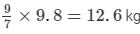Question 12: The ratio of the income to the expenditure of a family is 7 : 6. Find the saving if the income is Rs 1400.

ANSWER: The ratio of the income to the expenditure of a family is 7 : 6.

∴ Ratio of saving to the income = (7 − 6) : 7 = 1 : 7   (Saving = Total income −- Expenditure)
∵ Income of the family = Rs. 1400

∴ Saving = 1400 × 17 = Rs. 200

Question 13: The ratio of story books in a library to other books is 1 : 7. The total number of story books is 800. Find the total number of books in the library.

The ratio of story books in a library to other books is 1 : 7.
Out of (1 + 7) = 8 books, 1 book is a story book
Therefore,
When number of story books is 1, the total number of books = 8
When number of  story books is 800, the total number of books = 8 × 800
= 6400

Offer running on EduRev: Apply code STAYHOME200 to get INR 200 off on our premium plan EduRev Infinity!

103 docs

,

,

,

,

,

,

,

,

,

,

,

,

,

,

,

,

,

,

,

,

,

,

,

,

,

,

,

,

,

,

;